# How to find weight load on each car tyre during cornering?

• Automotive
• Sprotz
In summary, the speaker is looking for a way to simulate vehicle spin out or oversteer by manually calculating wheel load during cornering. They know two equations for calculating load during deceleration and cornering, but they are unsure of how to combine them to determine the weight transfer on each wheel. They are seeking a simple solution for simulation purposes.

#### Sprotz

So far I have not found a satisfactory answer anywhere on the internet for this one. I have read the "race car suspension class" section but could not find the bit I am interested in. I am trying to simulate vehicle spin out / oversteer by reducing traction on the rear wheels when cornering. My physics engine does not automatically calculate wheel load when cornering so I have to do it myself. I know two separate equations that calculate load on the wheels. One for longitudnal deceleration when braking, and one for lateral deceleration when cornering.
For a stationary vehicle, the wheel load on each axle is calculated as: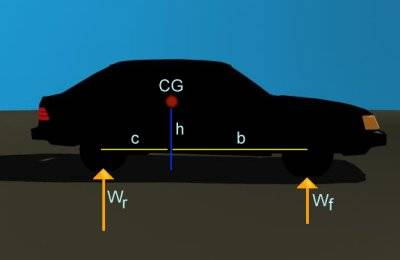Wf=(c/L)*W for the front axle
Wr=(b/L)*W for the rear axle
where c is the distance between the center of gravity and the rear axle, b is the distance between the center of gravity and the front axle, L is the wheelbase and W is the weight of the vehicle.
So during deceleration, the weight transfer on each axle is given by
Wf=(c/L)*W - (h/L)*M*a for the front axle
Wr=(b/L)*W + (h/L)*M*a for the rear axle
where h is the height of the center of gravity, M is the mass of the vehicle and a is acceleration/deceleration.
So the weight on each tyre for a stationary car would be Wf/2 for each front tyre and Wr/2 for each rear tyre, since the weight is distributed equally to these tyres if the center of gravity is at the half way point of the track width.
And the separate equation for weight transfer to the wheels during cornering, this is the equation for weight transfer for lateral deceleration is given by: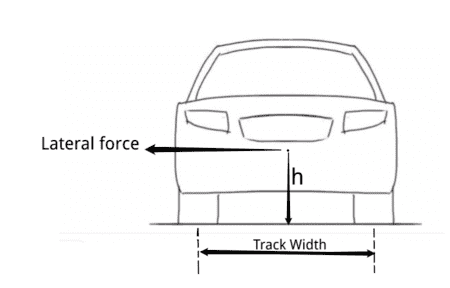Weight transfer=(Lateral deceleration/g)*W*h/Track width
Now how do I combine the two equations to give an equation that gives the weight transfer on each of the four wheels during both braking and cornering? So that I get the weight on each wheel instead of just on each axle?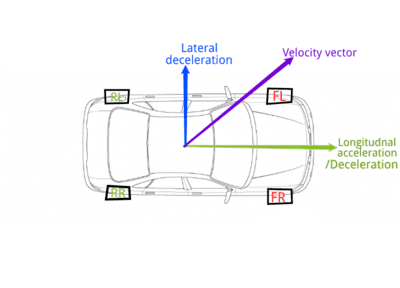Say, the car is both cornering and decelerating, and I simply got the longitudnal weight transfer divided by 2 on the front left tyre (FL) and added it to the lateral weight transfer divided by 2, would it be correct?

Or if I shifted the weight along the velocity vector by distance depending on deceleration and got the load distribution on each tyre as inversely proportional to the area of rectangle encompassing the wheel as shown below, where load on the front right tyre is inversely proportional to area A1. Same for the other tyres.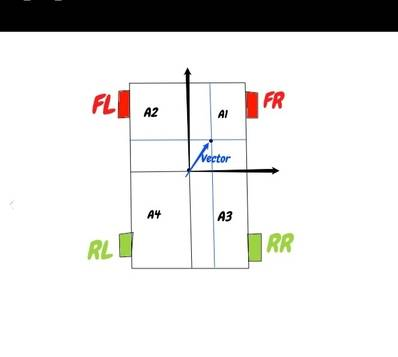The above assumes that there is no suspension for simplicity. So is any of the above solutions correct or is there another way of determining the load on each wheel? I need the simplest possible solution that is just enough to simulate spin out and I don't need a too realistic model, and please I prefer you answer if you know the solution, or a link to it as in my previous posts some people gave comments without really answering, or gave answers like "it's complicated" or "how can you calculate this without calculating that".
Or if you know Unity's vehicle physics engine, explain how to add spin out.

#### Attachments

Last edited:
I worked on simulations that included approximate ground handling of airplanes. In my case, the reason that I can not give you an answer is that we approached it completely differently. We calculated the equations of motion under the influence of all forces, including springs, shock absorbers, brakes, side force on tires, etc., at each time step and used the accelerations and velocities (both linear and rotational) to determine the positions a tiny time step later. That gave the new spring compressions, damping forces, etc for the next time step. Even then, we used many approximations and simplifications. I believe that is what is necessary for a relatively accurate answer. If you are only interested in something that will seem reasonable rather than accurate, you can probably use much simpler methods, but it sounds like you want accurate answers.

Last edited:
Sprotz said:
So far I have not found a satisfactory answer anywhere on the internet for this one. I have read the "race car suspension class" section but could not find the bit I am interested in. I am trying to simulate vehicle spin out / oversteer by reducing traction on the rear wheels when cornering. My physics engine does not automatically calculate wheel load when cornering so I have to do it myself. I know two separate equations that calculate load on the wheels. One for longitudnal deceleration when braking, and one for lateral deceleration when cornering.
For a stationary vehicle, the wheel load on each axle is calculated as:
View attachment 228787
Wf=(c/L)*W for the front axle
Wr=(b/L)*W for the rear axle
where c is the distance between the center of gravity and the rear axle, b is the distance between the center of gravity and the front axle, L is the wheelbase and W is the weight of the vehicle.
So during deceleration, the weight transfer on each axle is given by
Wf=(c/L)*W - (h/L)*M*a for the front axle
Wr=(b/L)*W + (h/L)*M*a for the rear axle
where h is the height of the center of gravity, M is the mass of the vehicle and a is acceleration/deceleration.
So the weight on each tyre for a stationary car would be Wf/2 for each front tyre and Wr/2 for each rear tyre, since the weight is distributed equally to these tyres if the center of gravity is at the half way point of the track width.
And the separate equation for weight transfer to the wheels during cornering, this is the equation for weight transfer for lateral deceleration is given by:
View attachment 228788
Weight transfer=(Lateral deceleration/g)*W*h/Track width
Now how do I combine the two equations to give an equation that gives the weight transfer on each of the four wheels during both braking and cornering? So that I get the weight on each wheel instead of just on each axle?
View attachment 228789
Say, the car is both cornering and decelerating, and I simply got the longitudnal weight transfer divided by 2 on the front left tyre (FL) and added it to the lateral weight transfer divided by 2, would it be correct?

Or if I shifted the weight along the velocity vector by distance depending on deceleration and got the load distribution on each tyre as inversely proportional to the area of rectangle encompassing the wheel as shown below, where load on the front right tyre is inversely proportional to area A1. Same for the other tyres.
View attachment 228790
The above assumes that there is no suspension for simplicity. So is any of the above solutions correct or is there another way of determining the load on each wheel? I need the simplest possible solution that is just enough to simulate spin out and I don't need a too realistic model, and please I prefer you answer if you know the solution, or a link to it as in my previous posts some people gave comments without really answering, or gave answers like "it's complicated" or "how can you calculate this without calculating that".
Or if you know Unity's vehicle physics engine, explain how to add spin out.
In your equation to determine the weight transfer (during longitudinal deceleration), it seemed like more weight acts on the rear axle than on the front axle when the breaks are applied.
But I think its wrong because the decelerating force is applied to the wheels by the ground but the centre of gravity of the vehicle will try to oppose it due to inertia.So the weght will act more on the front wheels than on the rear wheels.
If you substitute a negative valu for acceleration in the equation(since it is deceleration) then it is right.
Similarly for lateral deceleration, if you turn right, more weight act on the front left than the right.
This answer might even be silly.I am sorry if I have annoyed you.

Last edited:
Mohankpvk said:
In your equation to determine the weight transfer (during longitudinal deceleration), it seemed like more weight acts on the rear axle than on the front axle when the breaks are applied.
Since ##a## would be negative when brakes are applied, I think that the equations would have more weight on the front.

•Mohankpvk
FactChecker said:
Since ##a## would be negative when brakes are applied, I think that the equations would have more weight on the front.
But in the fourth image uploaded, it seems the vehicle turns right.But the maximum load seems to act on the front right wheel(since it was mentioned that load is inversly propotional to the area of the rectangle).I think it(max load) should act on the front left.

Mohankpvk said:
But in the fourth image uploaded, it seems the vehicle turns right.But the maximum load seems to act on the front right wheel(since it was mentioned that load is inversly propotional to the area of the rectangle).I think it(max load) should act on the front left.
Sorry ,its my mistake.The equations are right.Now I understood the drawing.

You are on the right track. The only thing you are missing is the F/R distribution of the lateral weight transfer.

For the longitudinal weight transfer, you correctly estimated that the weight transfer will be split 50/50 between the left and right wheels. You have to make an equivalent estimate for the lateral weight transfer between the front and rear wheels. This split can be anything between 100/0 and 0/100, according to the vehicle characteristics, mostly the suspension design. This ratio can often vary dynamically and even at the driver's command for some race cars. But you can certainly make an educated guess to simplify everything.

Case 1: No Suspension

If you have no suspension (ex.: a go-kart), the weight transfer should split between F/R axles according to the CG position, just like you instinctively done for the longitudinal acceleration. The CG is the middle, then you split 50/50; the CG is more toward one side than the other, then more weight transfer goes on that side and less on the other. The CG is dead on one axle, then that axle takes the full weight transfer. It is a simple lever arm equation, like your Wf=(c/L)*W equation.

CASE 2: With Suspension

In addition to the CG location, the weight transfer tends to go to the stiffest axle, roll resistance-wise. Let's illustrate an extreme case, this antique tractor: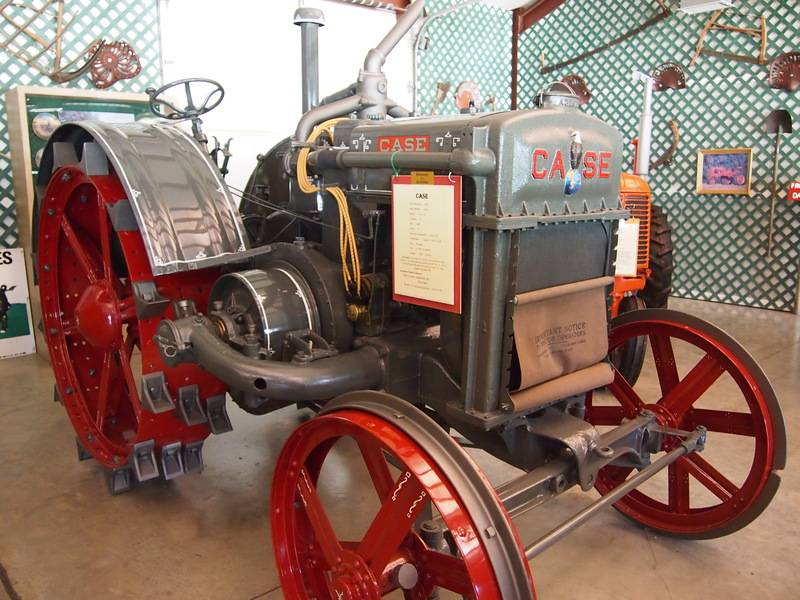The particularity of this suspension geometry is that the front axle not only doesn't have springs, it has no roll resistance whatsoever. The solid front axle is pinned at the center to the tractor's frame and, if the front end was in the air, anyone could move the wheels up & down by hand. This particular model probably has a solid rear axle with no springs, but even if it had rear springs, any lateral weight transfer have to go towards the rear end because the front can't take it; Thus you would have a F/R lateral weight transfer distribution of 0/100.

In more complex suspension, the stiffness of the springs, the anti-roll characteristics of the suspension geometry, anti-roll bar stiffness and even frame torsion resistance all influence which end is stiffer than the other. If both ends have the same roll resistance (and the CG is half-way between both axles), then the split will be 50/50. Fine tuning is done to achieve the perfect balance for what is wished to be accomplished for a particular car and - for race cars - even on a particular track.

But you can «guesstimate» the split ratio rather easily for simplification. There are no wrong answers, only wrong designs.

#### Attachments

What is your application for the results of your calculations? It clearly cannot be related to actual "on road" vehicles due to the suspension factors discussed above plus plus tire angle effects etc. On the other hand, if it for a video game or general demonstration of the spinout phenomenon under different acceleration conditions then the suspension factors can be ignored and vehicle simply treated as a rigid structure with a wheel mounted at each corner (i.e. go cart) and your current formulas can be utilized by summing as you propose. Once you have done that, you will still be required to set your own criteria for what combination of your load transfer results represent a vehicle oversteer spinout point under each road condition dry, wet, ice, mud, etc. if that is a variable in your application.

•FactChecker and Sprotz
So summing up the results of the two equations would be sufficient? Okay then, unless someone comes up with a different method.
All I want is to simulate car physics in a game, with spin out.

For a game accuracy of the simulations is not as important as having reliable and repeatable vehicle responses for all players that keeps the playing field level for everyone.

•FactChecker